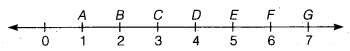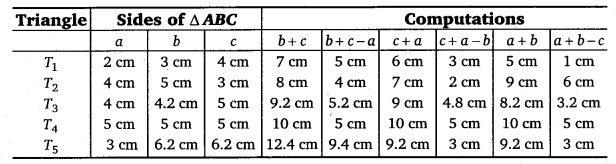# Class 6 Maths NCERT Solutions for Chapter 5 Understanding Elementary Shapes Ex – 5.1

## Understanding Elementary Shapes

Question 1.
What is the disadvantage in comparing line segments by mere observation?

Solution:
The disadvantage in comparing line segments by mere observation is the inaccuracy in judgement.

Question 2.
Why is it better to use a divider than a ruler, while measuring the length of a line segment?

Solution:
It is better to use a divider with ruler as it given an accurate measurement of the line segment.

Question 3.
Draw any line segment, say AB. Take any point C lying in between A and B. Measure the lengths of AB, BC and AC. Is AB = AC + CB?

[Note: If A, B, C are any three points on a line, such that AC + CB = AB, then we can be sure that C lies between A and B.]

Solution:
On measuring the lengths of line segments AB, BC and AC using ruler, we findAB =6.5 cm,
CB = 4.2 cm,
and AC = 2.3 cm
Now, AC +CB = 2.3 cm +4.2 cm = 6.5 cm = AB
AB = AC+CB.

Question 4.
If A, B, C are three points on a line such that AB = 5 cm, BC = 3 cm and AC = 8 cm, which one of them lies between the other two?

Solution:
Since, AB + BC = 5 cm +3 cm = 8 cm = AC
∴ A, B, C are collinear and B lies between A and C.

Question 5.
Verify, whether D is the mid point of$\overline { AG }$.Solution:
Since AD = AB +BC + CD = 3 units
and, DG = DE +EF + FG = 3 units
∵ AD = DG [∵ Each = 3 units]
Thus, D is the mid-point of$\overline { AG }$.

Question 6.
If B is the mid point of$\overline { AC }$ and C is the mid point of$\overline { BD }$, where A, B, C, D lie on a straight line, say why AB = CD?

Solution:
∵ B is the mid point of$\overline { AC }$. Therefore, AB = BC.
∵ C is the mid-point of$\overline { BD }$. Therefore, BC = CD.
Thus, AB =BC = CD i.e., AB = CD.

Question 7.
Draw five triangles and measure their sides. Check in each case, if the sum of the lengths of any two sides is always less than the third side.

Solution:
Draw any five triangles, T1, T2, T3, T4 and T5. label each one as ∆ ABC. Measure, in each case, the three sides a = BC, b = CA and c = AB.Let us tabulate the measurements of sides as under:From the above table, we find that
(i) each value of b + c – a is positive;
(ii) each value of c + a – b is positive;
(iii) each value of a + b – c is positive.
Now, b + c – a is positive ⇒ b + c – a > 0 ⇒ b + c > a
c + a – b is positive ⇒ c + a- b > 0 ⇒ c + a > b
a+ b – c is positive ⇒ a + b – c > 0 ⇒ a + b > c
Thus, it is verified that the sum of any two sides of a triangle is greater than the third side.
∴ The statement ‘the sum of two sides is ever less than the third side’ is never true.